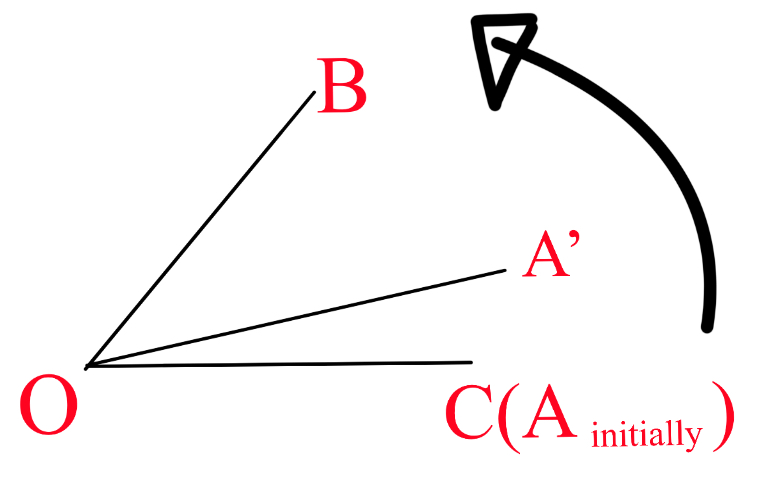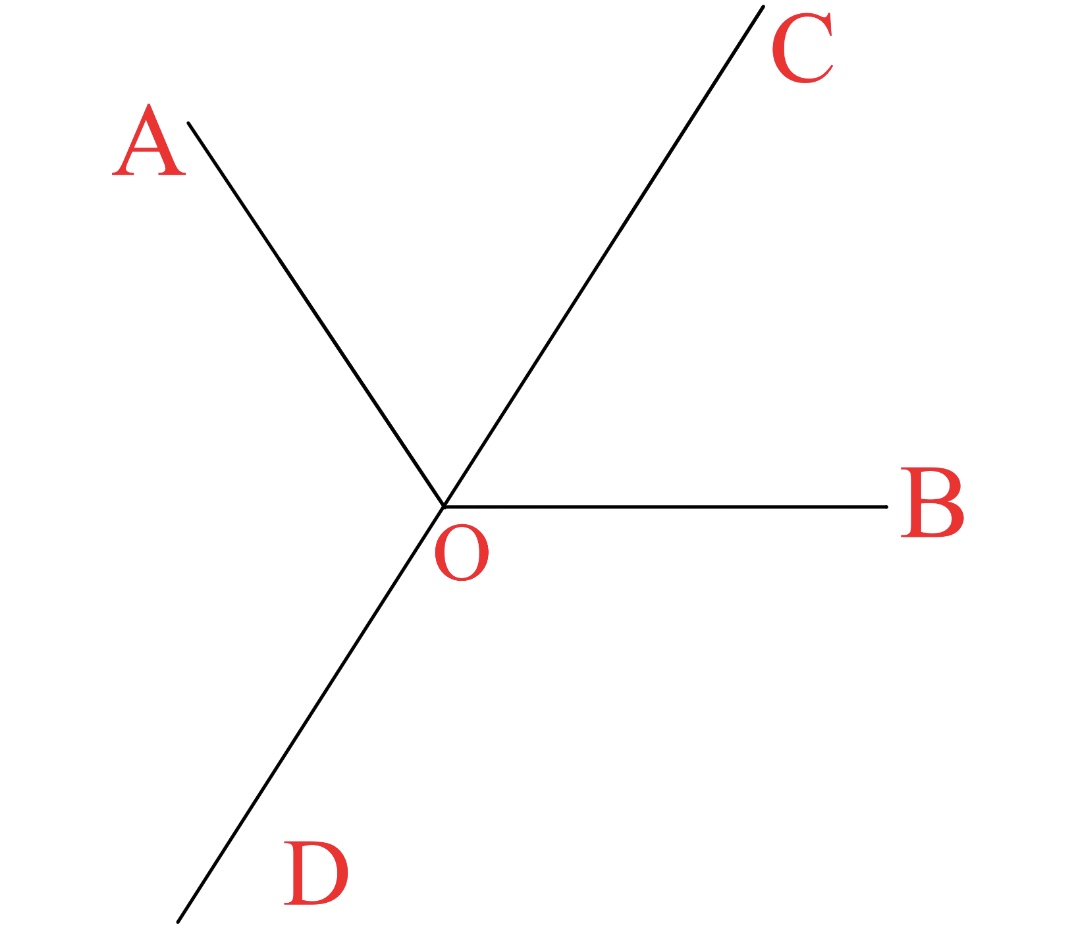# Can be solved by most 13-year-olds in China‘-2

Geometry Level 3

Sorry that the question seems confusing at first, since brilliant glitched and didn’t save my edits :/

Whole set
$\small \color{#D61F06} \text{Carefully read assumptions before answering}$
As in the figure, there are rays $OA,OB,OC$. $\angle BOC=50^\circ$. Let $OA$ be a rod which overlaps ray $OC$. As ray $OA$ rotates counter-clockwise around point $O$ at a speed of $10^\circ$ per second, there are moments when one of $OA,OB,OC$ bisects the angle formed by the other two.It rotates COUNTER-CLOCKWISE :)

Denote time as $t$ in seconds. $0\le t\le 36$. If above scenario happens at $t=1,t=2,t=3$, submit your answer as the product of all possible values of $t$, in this example submit $1\times 2\times 3=6$.

Assumptions:

• There is no angle greater than $180^\circ$ or less than $0^\circ$. No angle equals $180^\circ$ or $0^\circ$.
• This must be explained further:• Here $OC$ bisects $\angle AOB$ while $OD$ is not considered as a bisector at all.
• Angle bisectors are rays. Rays, straight lines and line segments are to be STRICTLY DISTINGUISHED FROM ONE ANOTHER.
×